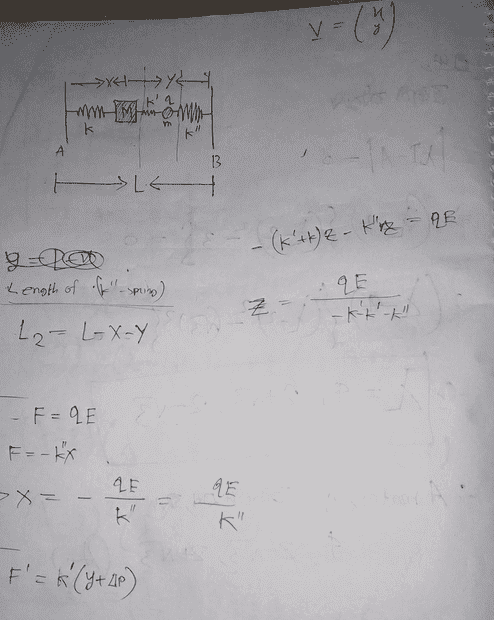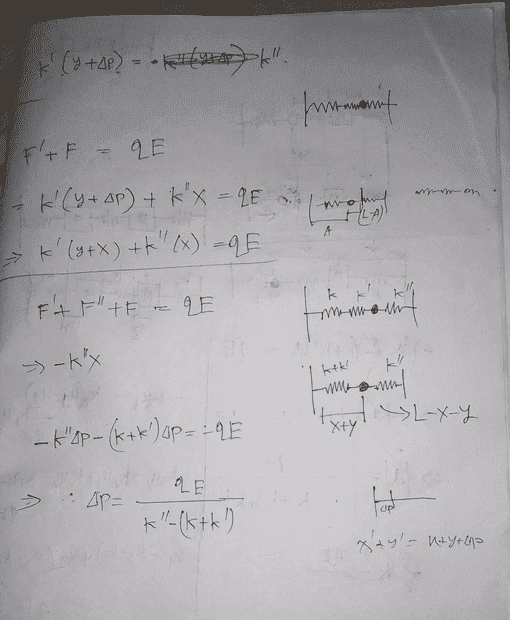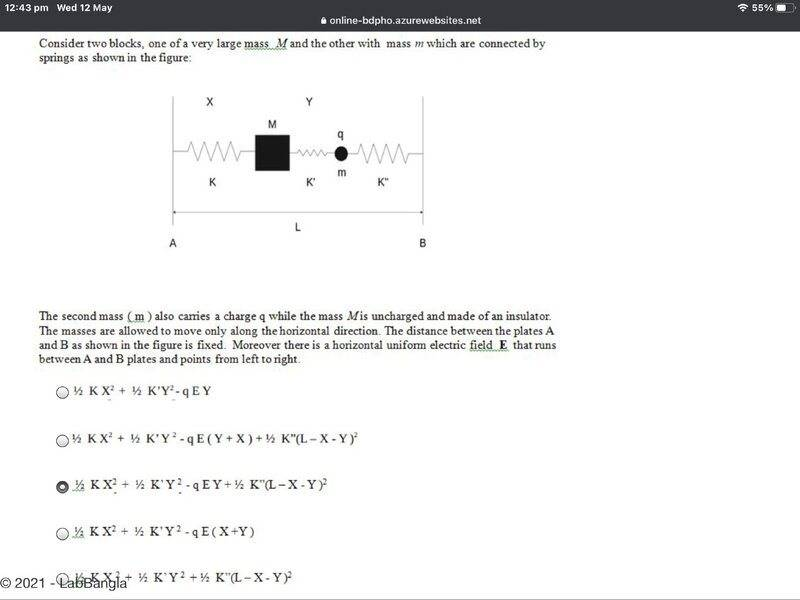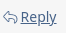# Find the electric field

Homework Statement:
Electric field
Relevant Equations:
F=kq/r^2
Solution attempt#### Attachments

berkeman
Mentor
Find the electric field where? Can you post the full problem statement, and maybe the figure that is in the problem sheet that you are working from? Thanks.

Delta2
Homework Helper
Gold Member
Seems to me you don't like to write a lot. Maybe english is not your mother language ok.

The attachment explains the problem setup but then it offers some choices but it doesn't mention what is the question. It looks like it is asking what is the total potential energy of the system.

And besides that, on your own work you seem to work towards finding the equilibrium position for charge q. Is that what you trying to find?

Seems to me you don't like to write a lot. Maybe english is not your mother language ok.

The attachment explains the problem setup but then it offers some choices but it doesn't mention what is the question. It looks like it is asking what is the total potential energy of the system.

And besides that, on your own work you seem to work towards finding the equilibrium position for charge q. Is that what you trying to find?
Yes! It is. That's what I wrote. I was trying to find that value. Potential energy depends on the X²

•AF Fardin
Yep

Delta2
Homework Helper
Gold Member
Well I still don't understand your work.

At start you write ##F=qE## or ##k''x=qE##
But after some point you write ##F'+F=qE##
And later ##F''+F'+F=qE##
only one case can be true, or I just don't understand something here.

•AF Fardin
berkeman
Mentor
Yep

I was about to delete your reply as unnecessary, but when I checked your previous posting history, I see that you posted about a similar/same problem earlier today and the post was deleted because you showed no effort. Your attachment is added below; are you and @Istiakshovon in the same class?#### Attachments

•Delta2
Steve4Physics
Homework Helper
Gold Member
Hi @Istiakshovon. I will also chip in.

Title: Find the electric field”
The question is apparently not about finding the electric field!

Homework Statement: Electric field”
That’s not a homework statement. You need to give the complete question.

Relevant Equations: F = kq/r^2
The equation is wrong. Maybe you mean E = kq/r^2 or F = kq₁q₂/r^2. However, neither of these equations is relevant to your question!

Like @Delta2, I too I can’t understand your solution attempt.

You have ignored @berkeman's post (#2). Is there a reason? I don’t think you will get help unless you at least post the complete question, as requested. (I can possibly guess what the question is but I might be wrong.)

I was about to delete your reply as unnecessary, but when I checked your previous posting history, I see that you posted about a similar/same problem earlier today and the post was deleted because you showed no effort. Your attachment is added below; are you and @Istiakshovon in the same class?

View attachment 284973
Actually, he was new to the community. He didn't understand how the community works. He wasn't familiar with these tools. So, he told me to create new thread here cause, I had talked about the community to him. Then, I had posted it. I hope you understood. I know that "Yep" comment is unnecessary. So, you can delete that. He doesn't know code of conduct of the community either. I haven't read COC also.

Hi @Istiakshovon. I will also chip in.

Title: Find the electric field”
The question is apparently not about finding the electric field!

Homework Statement: Electric field”
That’s not a homework statement. You need to give the complete question.

Relevant Equations: F = kq/r^2
The equation is wrong. Maybe you mean E = kq/r^2 or F = kq₁q₂/r^2. However, neither of these equations is relevant to your question!

Like @Delta2, I too I can’t understand your solution attempt.

You have ignored @berkeman's post (#2). Is there a reason? I don’t think you will get help unless you at least post the complete question, as requested. (I can possibly guess what the question is but I might be wrong.)
As I mentioned in my previous reply that this question is not mine. It's another person's. I didn't have much more time to look at his works. So, that what I simply understood without reading answer and question, I had just added that. As I said I didn't have much more time, that's why I added unnecessary **Homework Statement**

I was trying to edit my question. Unfortunately, there's no edit button. Is it bug? The thread was migrated from Calculus and Physics. I think that's why I don't have anymore access to edit the question.

Delta2
Homework Helper
Gold Member
I was trying to edit my question. Unfortunately, there's no edit button. Is it bug?
No it isn't bug, the forum software doesn't let you edit the post a few hours after it was initially posted.

No it isn't bug, the forum software doesn't let you edit the post a few hours after it was initially posted.
So, How can I edit Homework Statement?

Delta2
Homework Helper
Gold Member
Just post a new message here with the full homework statement. Later when a moderator (for example @berkeman) might see your new message, he will copy paste the homework statement to the old message.

•Istiakshovon
Homework Statement: The second mass (m) also carries a charge q while the mass M is uncharged and made of an insulator. The masses are allowed to move only along the horizontal direction. The distance between the plates A and B as shown in the figure is fixed. Moreover there is a horizontal uniform electric field E that runs between A and B plates and points from left to right.

https://www.physicsforums.com/attachments/e4e2c5e8-5062-4bbf-94a7-55bbd56e393c-jpeg.284961/

Delta2
Homework Helper
Gold Member
Homework Statement: The second mass (m) also carries a charge q while the mass M is uncharged and made of an insulator. The masses are allowed to move only along the horizontal direction. The distance between the plates A and B as shown in the figure is fixed. Moreover there is a horizontal uniform electric field E that runs between A and B plates and points from left to right.

https://www.physicsforums.com/attachments/e4e2c5e8-5062-4bbf-94a7-55bbd56e393c-jpeg.284961/
Ok fine for the description of the problem setup, but you didn't tell us what is the question asked. Is it "Find the equilibrium position for mass m"?

Ok fine for the description of the problem setup, but you didn't tell us what is the question asked Is it "Find the equilibrium position for mass m"?
I am forwarding it to @AFFardin. @AFFardin Reply to him using the reply button to his replyWell I still don't understand your work.

At start you write ##F=qE## or ##k''x=qE##
But after some point you write ##F'+F=qE##
And later ##F''+F'+F=qE##
only one case can be true, or I just don't understand something here.
F"+F'+F=qE
This is true I think !!!!

F"+F'+F=qE
This is true I think !!!!
At First, I wasn't sure about it!
I was trying and thinking with some roughs!!
And that's what made you confused. My final thought is this (F"+F'+F=qE).
Still don't know that my approach is correct or wrong!

•Delta2
I was about to delete your reply as unnecessary, but when I checked your previous posting history, I see that you posted about a similar/same problem earlier today and the post was deleted because you showed no effort. Your attachment is added below; are you and @Istiakshovon in the same class?

View attachment 284973
Yes

Ok fine for the description of the problem setup, but you didn't tell us what is the question asked. Is it "Find the equilibrium position for mass m"?
[6/25, 09:49] AF_Fardin: Both X and Y
[6/25, 09:49] AF_Fardin: Means for both masses

Delta2
Homework Helper
Gold Member
ok so you want the positions of the two masses for when the whole system is in equilibrium. Hold on while I work on my reply (might take a while cause I am a bit busy with other things at the moment.)

Delta2
Homework Helper
Gold Member
Ok I think the correct system of equations is
$$Kx-K'y=0$$
$$K'y+Eq-K''z=0$$
$$x+y+z=L$$
The first equation is from the equilibrium condition on mass M, the second from the equilibrium condition on mass m, and the third is self explanatory

P.S We assume that the springs have zero natural length.

Ok I think the correct system of equations is
$$Kx-K'y=0$$
$$K'y+Eq-K''z=0$$
$$x+y+z=L$$
The first equation is from the equilibrium condition on mass M, the second from the equilibrium condition on mass m, and the third is self explanatory

P.S We assume that the springs have zero natural length.
Wow thank you !!
Could you please explain it explicitly for me?

Ok I think the correct system of equations is
$$Kx-K'y=0$$
$$K'y+Eq-K''z=0$$
$$x+y+z=L$$
The first equation is from the equilibrium condition on mass M, the second from the equilibrium condition on mass m, and the third is self explanatory

P.S We assume that the springs have zero natural length.
But, actually your approximation doesn't match with any option. What should I do next (with this equation)?